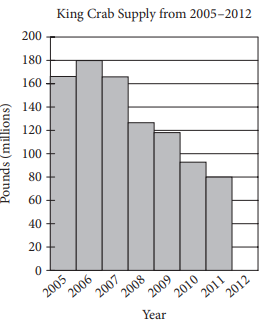mycollegehive
The graph above shows the supply, in millions of pounds, of...
PSAT PSAT-math-test math0The graph above shows the supply, in millions of pounds, of king crab harvested and sold from 2005 to 2011. The information for the year 2012 is not included in the graph.

In 2011, the price of king crab was \$17 per pound. In 2012, x million pounds of king crab were sold at \$16 per pound. If the total money generated from sales each year was the same, what is the value of x ?

232 viewsShareFollowSchool not set Nigeria
11 January 2021University of Benin Nigeria
04 February 20210According to the graph, the king crab supply in 2011 was 80 million pounds.

So at the price of \$17 per pound, the revenue generated, in millions of dollars, from the sales of king crab in 2011 was (80)(17) = 1360.

Since x millions pounds of king crab was sold in 2012 at the price of \$16 per pound, the revenue in 2012 was 16x million dollars.

It is given that the revenue generated from the sales of king crab in 2011 was the same as the revenue in 2012.

Therefore, 16x = 1360, so x = 85.Share

### Groups

How to insert math formulas/equations### Related Tags

PSAT

3 followers

94 questionsPSAT-math-test

4 followers

94 questionsmath

23 followers

1262 questions